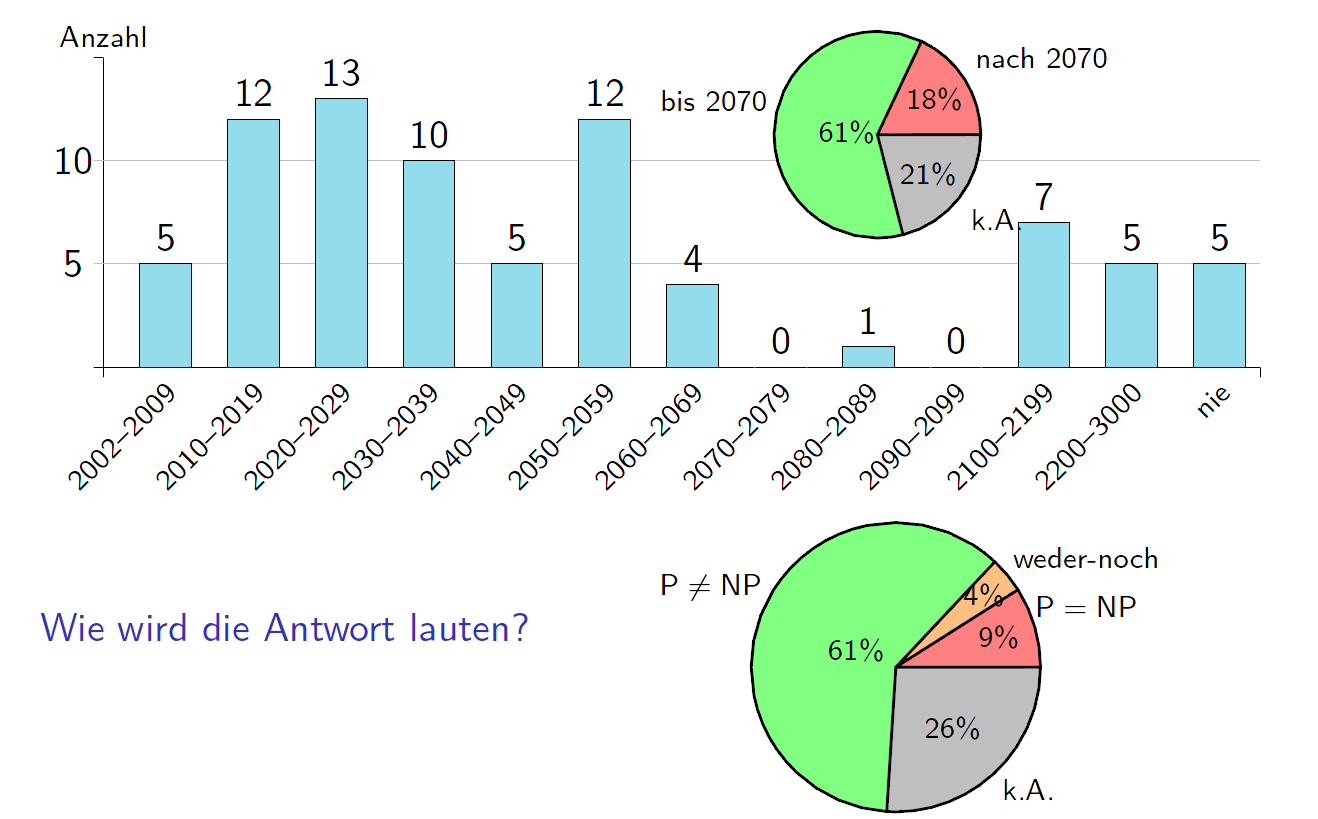## Wednesday 6 November 2013

### (No) Problem

Today, I would like to talk about an interesting problem.

First of all, we have a class that includes all the solutions to problems that can be solved efficiently (polynomial time). Let us call this class P. The class of questions for which an answer can be verified in polynomial time is called NP.

The most difficult problems in NP are called NP-complete. An example:

Imagine there is a group of young adolescents at a round table. Some of them know eachother, some do not. Is it possible to find an order where two people sit next to eachother so that they are happy with the situation? (They are happy if they know eachother.)

While there are many algorithms to this problem, there is no real solution. Real solution:= efficient solution:= not exponential.

One thing we know for sure is, that if everyone is already sitting, it is easy to determine who likes whom. But finding the right seat plan is difficult.

Another one from wikipedia:

Consider the subset sum problem, an example of a problem that is easy to verify, but whose answer may be difficult to compute. Given a set of integers, does some nonempty subset of them sum to 0? For instance, does a subset of the set {−2, −3, 15, 14, 7, −10} add up to 0? The answer "yes, because {−2, −3, −10, 15} adds up to zero" can be quickly verified with three additions. However, there is no known algorithm to find such a subset in polynomial time (there is one, however, in exponential time, which consists of 2n-1 tries), and indeed such an algorithm can only exist if P = NP; hence this problem is in NP (quickly checkable) but not necessarily in P (quickly solvable).

Now, at this point you are either still here or closed the window long ago. If you are still here, you probably ask. Why should I care?

Well, for an example, because you can get rich. The NP problem is one of the seven Millennium Prize Problems selected by the Clay Mathematics Institute to carry a US\$1,000,000 prize for the first correct solution. You get the money if you find correct proof, or if you can prove that there is no solution.

Or, if you have no interest in money, maybe you would be happy about a fix prof. position at Harvard or MIT.

Either way, there is one more interesting thing to this problem. There is a statics from 2002, where experts at this field had a survey. The question was: "Will there be ever a solution, and if yes, when?"As you can see, most experts believe that it will be solved soon. Some of them already failed with their predictions. I believe that it either will be solved in 2040-2070 or never. But this is just my oppinion, let us hear yours.

If you have further questions or perhaps a solution, let us know. (If you have a solution, perhaps you better not tell me ;) )